.EntradaApresentaçãoLeitores e visitantesCursos e PalestrasNossa empresaOportunidades• Cadastre-se!DivulgaçãoA Coleção• O CD Números• COMPRAReportagens• Mathematica 9• CramerSarrus 2• playEquationCurso para ANPADCurso para ANPADCondecorações / Awards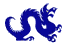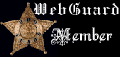## Counting Lattice Points with GrafEq

By Carlos César de Araújo - cca@gregosetroianos.mat.br

Portuguese version (with different examples)

We will see on this page how to use GrafEq to count lattice points (i.e., points with integer coordinates) in certain subsets of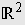. Let us first consider a disk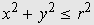. It is not dificult to show that the number of lattice points in this region is given by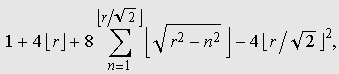where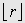is the floor of r. Thus there are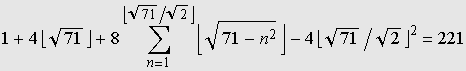pairssuch that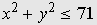.

We can use GrafEq to visualize this result. All we have to do is to enter with the appropriate set of relations in the algebraic relation window. Equational conditions that characterizes the set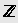are easy to discover; two of them are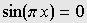and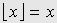. The animation below shows the scanning process used by GrafEq to select the lattice points. You can check that the last frame ends with 221 points. (Press the Escape key to stop the GIF animation.)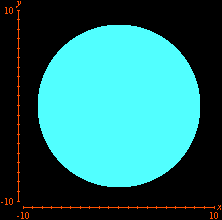Additional constraints can also be included. Below we see the selection of pointsin the previous disk for which x and y are relatively prime.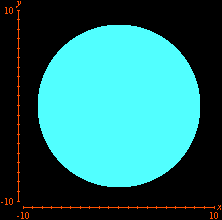This time we are left withlattice points. The real answer, nevertheless, is 136. GrafEq missed the points. What happened? This has to do with the current implementation of gcd in GrafEq 2.11 (the latest version), according to whichis undefined if. Jeff Tupper will fix this behavior in a future release.

Looking for lattice points in curves is the subject of Diophantine equations. The next animation shows how GrafEq detects the integer solutions to the equationThe graph reveals five lattice points on the hyperbola, namely,. (Of course, the pointmust be excluded.) It is not difficult to prove that these are the only integer solutions.

Carlos César de Araújo,  August 12, 2002ProvasLaboratóriosConceitosErros MatemáticosHistóriaLógicaEducaçãoÁlgebraCálculoNúmerosEscrita matemáticaGeometria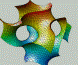SoftwaresTHEOREMA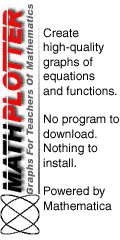instruções